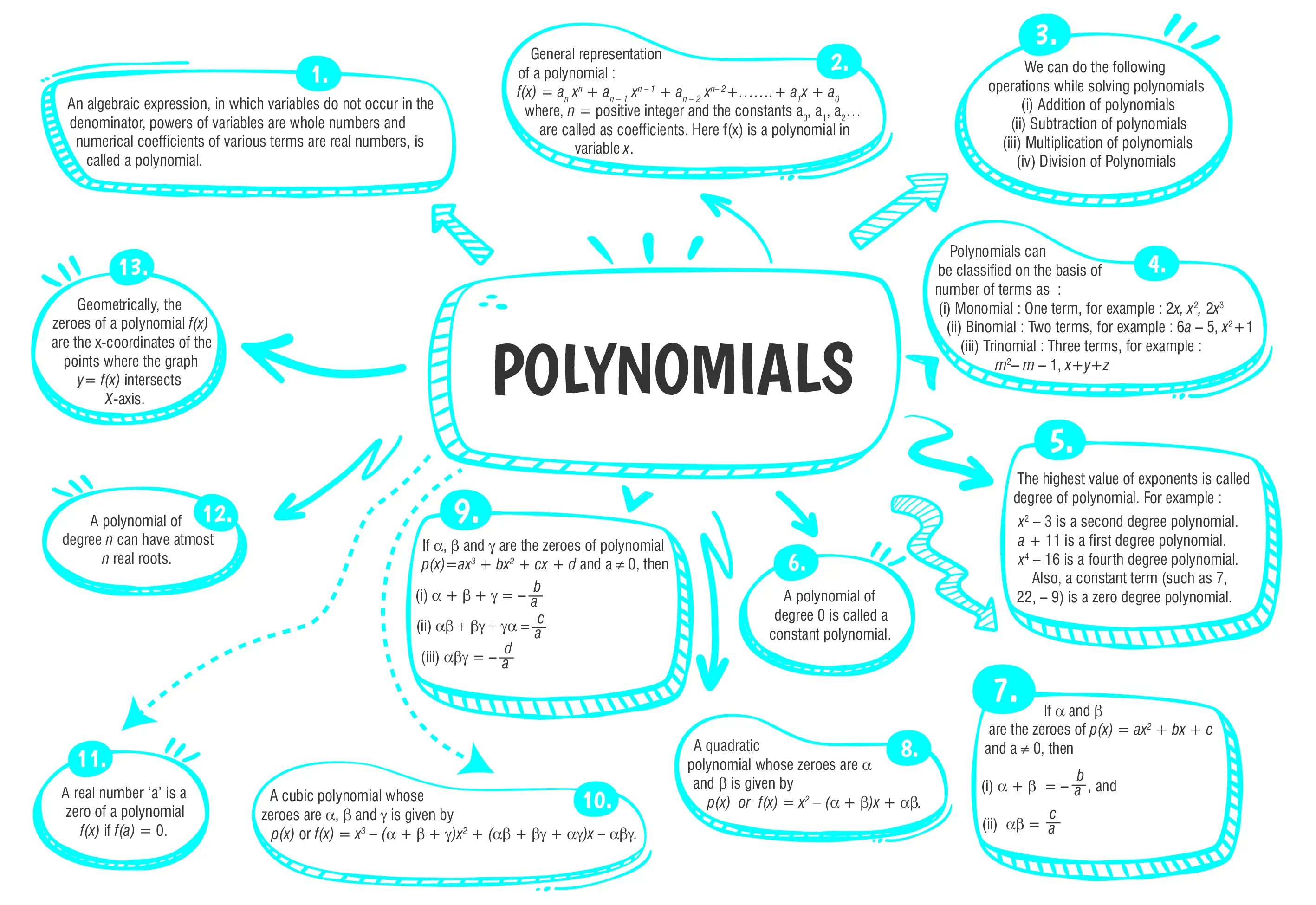Polynomials

# Class 10 Maths Chapter 2 Polynomials Important QuestionsHere are some essential Class 10 Mathematics questions for Chapter 2, Polynomials. These carefully curated questions aim to assist students in their preparation for the CBSE Class 10 Mathematics Examination 2023-24. Practicing a diverse range of question types will not only help students clarify their doubts but also enable them to prepare more effectively for the exams. By solving these questions, students can build confidence in their problem-solving skills and enhance their overall performance in the Polynomials chapter.

## Introduction

In Chapter 2 of Class 10 Mathematics, Polynomials you will learn about polynomials, their types, and the concept of degree. Additionally, we will study the zeros of a polynomial and the relationship between zeros and coefficients of quadratic polynomials.

### What are Polynomials ?

A polynomial is a mathematical expression made up of variables (usually represented by the letters x, y, etc.), coefficients (real numbers), and non-negative integer exponents. The general form of a polynomial is given by:
P(x) = anxn + an-1xn-1 +an-2xn-2 + ………………. + a1x + a0

Where an, an-1, an-2, ……………………, a1, a0 are called coefficients of xn, xn-1, xn-2, ….., x and constant term respectively and it should belong to a real number.### Class 10 Polynomials Important Questions and Answers

#### (a) 10(b) -10(c) -7(d) -2

Ans. (b)

Explanation:
Given polynomial x2 + 3x + k
Also, given that 2 is one of the zeros of the polynomial
Let  p(x) = x2 + 3x + k
∵      x = 2 is a zero of p(x)
∴     p(2) =0
⇒    (2)2 + 3(2) + k = 0
⇒     4 + 6 + k = 0
⇒      10 + k = 0
⇒        k = -10

#### (a) -3,2(b) -3 -2(c) 3,2(d) 3, -2

Ans. (d)

Explanation:
f(x) = x2 + x - 6
= x2 - 3x + 2x - 6
= x(x - 3) + 2(x - 3)
= (x + 2)(x - 3)
To find zeros put f(x) =0
∴       x = -2, 3

#### Q 3. Solve for x:$$3x^2- 2\sqrt{6}x + 2 = 0$$

Ans. $$x= \sqrt{{\Large\frac{2}{3}}},\sqrt{{\Large\frac{2}{3}}}$$

Explanation:
$$Given, 3x^2- 2\sqrt{6}x + 2 = 0$$
$$⇒ 3x^2- \sqrt{6}x - \sqrt{6}x + 2 = 0$$
$$⇒ \sqrt{3}x(\sqrt{3}x - \sqrt{2} ) - \sqrt{2}(\sqrt{3}x - \sqrt{2} ) = 0$$
$$⇒ (\sqrt{3}x - \sqrt{2} )(\sqrt{3}x - \sqrt{2} ) = 0$$
$$⇒ x = \sqrt{\Large{\frac{2}{3}}},\sqrt{\Large{\frac{2}{3}}}$$

#### Q 4. Find the value of p so that the polynomial px(x - 3) + 9 = 0 has two equal roots.

Ans. p = 4

Explanation:
Given,
px(x - 3) + 9 = 0
or  px2 - 3px + 9 = 0
Let ⍺, β be the zeroes of the polynomial.
$$\text{Then, α + β = 3 and αβ =} {\Large\frac{9}{p}}$$
Also,             α = β
∴            2α = 3
$$\text{or \space \space α = β} = {\Large\frac{3}{2}}\\[3.5 bp] ∴ {\Large\frac{9}{4}} = {\Large\frac{9}{p}}$$
⇒                p = 4
Hence, the zeroes of the polynomial px(x - 3) + 9 = 0 will be equal when p = 4.

#### Q 5. Sum of zeroes of the polynomial 2x2 – 4x + 5 is 4. Navdeep at once said “it is false” Do you agree with Navdeep ? Justify.

Ans. Navdeep is right

Explanation:
Yes
On comparing the given equation with ax2 + bx + c,
We get
Here a = 2, b = -4, c = 5
$$\text{Sum of zeroes = }{\Large \frac{-b}{a}}={\Large\frac{-(-4)}{2}}={\Large\frac{4}{2}}= 2$$
∴ Navdeep is right.### CBSE Class 10 Maths Chapter wise Important Questions

Chapter No. Chapter Name
Chapter 1 Real Number
Chapter 2 Polynomials
Chapter 3 Pair of Linear Equations in Two Variables
Chapter 5 Arithmetic Progressions
Chapter 6 Triangles
Chapter 7 Coordinate Geometry
Chapter 8 Introduction to Trigonometry
Chapter 9 Some Applications of Trigonometry
Chapter 10 Circles
Chapter 11 Areas Related to Circle
Chapter 12 Surface Areas and Volumes
Chapter 13 Statistics
Chapter 14 Probability

#### Conclusion

The chapter on Polynomials helps students develop algebraic skills and prepares them for more advanced topics in higher classes. It also finds practical applications in various fields such as science, engineering, and economics. Understanding polynomials is crucial for building a strong foundation in algebra and problem-solving skills.

#### Q1: Differentiate between monomial, binomial, trinomial, and multinomial.

Ans: Monomial: A polynomial with only one term, such as 5x3, 2y, etc.

• Binomial: A polynomial with two terms, such as 3x + 2, 2y2 - 5, etc.
• Trinomial: A polynomial with three terms, such as 4x2 + 3x + 1, 2y3 - 6y2 + 7y, etc.
• Multinomial: A polynomial with more than three terms, such as 5x4 + 2x3 - 3x2 + 7x - 1, etc

#### Q2:  How do you find the degree of a polynomial? Explain with examples.

Ans: The degree of a polynomial is the highest power of the variable 'x' (or any other variable) in the expression. To find the degree, identify the term with the highest power of the variable.

#### Q3:  What is the Factor Theorem? How is it useful in finding factors and roots of polynomials?

Ans: Factor Theorem: If P(a) = 0, then (x - a) is a factor of the polynomial P(x).
The Factor Theorem helps in finding factors and roots of polynomials. If we know one root (zero) of a polynomial, we can easily find the corresponding factor. Additionally, it simplifies the process of polynomial factorization.

#### Q4:  State the degree of the zero polynomial.

Ans: The degree of the zero polynomial is undefined since it does not have any terms.

#### Q5:  If the degree of a polynomial is 'n,' what is the maximum number of roots it can have?

Ans: The maximum number of roots a polynomial of degree 'n' can have is 'n'.

###### Copyright 2022 OSWAL PUBLISHERS Simplifying ExamsPhone:  (+91) 78959 87722
Mail: support@oswalpublishers.in